## LetsPlayMaths.Com

WELCOME TO THE WORLD OF MATHEMATICS

# Class 6 Fractions

Introduction to Fractions

Type of Fractions

Proper Fractions

Improper Fractions

Mixed Fractions

Like Fractions

Unlike Fractions

Conversion of an Improper Fraction to Mixed Fraction

Conversion of a Mixed Fraction to Improper Fraction

Equivalent Fraction

Subtraction of Like Fractions

Subtraction of Unlike Fractions

Multiplication of Fraction with a Whole Number

Multiplication of Fractions

Reciprocal of Fraction

Division of Fractions

Fractions Test

Fractions Worksheet

## Introduction to Fractions

Fraction is a part of whole and it is denoted as pq. 'p' is known as numerator and 'q' is known as denominator. Denominator tells us about into how many equal parts the whole is divided. Numerator tells us how many parts are considered of the whole.

## Type of Fractions

Mainly there are five types of fractions.

1. Proper fractions

2. Improper fractions

3. Mixed fractions

4. Like fractions

5. Unlike fractions

## Proper Fractions

Fractions in which denominator is greater than numerator are known as proper fraction.

Example. 23, 35, 47 (Here all the numerators are less than denominators)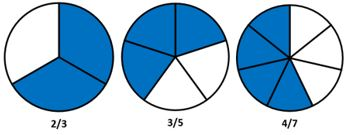## Improper Fractions

Fractions in which numerator is greater than or equal to the denominator are known as improper fractions.

Example. 32, 43, 97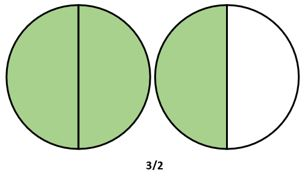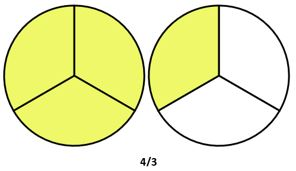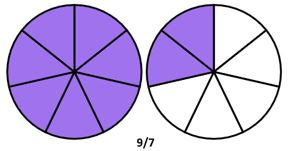## Mixed Fractions

A number with a whole number part and a fractional part is known as mixed fraction.

Example. 1 23, 112, 123

## Like Fractions

Fractions having same denominators are known as like fractions.

Example. 25, 35, 45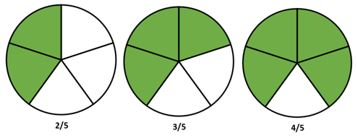## Unlike Fractions

Example. 23, 35, 47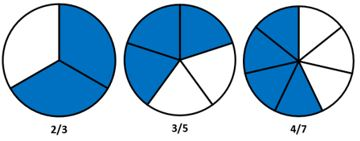## Conversion of an Improper Fraction to Mixed Fraction

Let's consider an improper fraction i.e. 75, now divide 7 by 5 as shown below.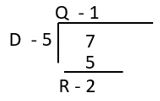D − Divisor

Q − Quotient

R − Remainder

7/5 = QRD = 125

## Conversion of a Mixed Fraction to Improper Fraction

WND = ((WxD)+N)D

W = Whole number

N = Numerator

D = Denominator

Let's see some examples.

Example 1. Convert 225 into improper fraction.

Solution. 225 = ((2x5)+2)5 = ((10)+2)5 = 125

Example 2. Convert 123 into improper fraction.

Solution. 123 = ((1x3)+2)3 = 53

## Equivalent Fraction

All fractions that have the same value are known as equivalent fraction. Let's see some examples of equivalent fractions.

12 = 24 = 48 = 816 = ...

23 = 46 = 812 = 1624 = ...

If the denominators of two or more fractions are same, then we add the numerators and keep the denominators as is. Let's see some examples.

Example 1. Add 25 and 45.

Solutions. 25 + 45 = (2+4)5 = 25

Example 2. Add 29 and 59.

Solutions. 29 + 59 = (2+5)9 = 79

If the denominators of two fractions are different, then we find the LCM of the denominators. Rewrite each fraction with LCM as common denominators and add the fractions as like fractions. Let's see some examples.

Examples 1. Add 25 and 37.

Solution. LCM of 5 and 7 is 35.

25 = 1435 and 37 = 1535

= 1435 + 1535
= (14+15)35 = 2935

Examples 2. Add 512, 716 and 9/24.

Solution. The LCM of 12, 16 and 24 is 48.

512 = 2048      716 = 2148      924 = 1848

2048 + 2148 + 1848

= (20+21+18)48 = 5948 = 11148

## Subtraction of Like Fractions

If the denominators of two fractions are same, then we subtract the numerators and keep the denominators as is. Let's see some examples.

Example 1. Subtract 37 from 57.

Solution. 5737 = (5−3)7 = 27

Example 2. Subtract 924 from 1324

Solution. 1324924 = (13−9)24 = 424 = 16

## Subtraction of Unlike Fractions

If the denominators of two fractions are different, then we find the LCM of the denominators. Rewrite each fraction with LCM as common denominators and subtract the fractions as like fractions. Let's see some examples.

Example 1. Subtract 13 from 45

Solution. LCM of 3 and 5 is 15.

13 can be written as (1x5)(3x5) = 515

45 can be written as (4x3)(5x3) = 1215

1215515 = (12−5)15 = 715

Example 2. Simplify 213 − 1348

Solution. 213 − 1348

First, we must convert the mixed fractions into improper fractions.

735148 (LCM of 3 and 48 is 48)

= 112485148 = (112−51)48 = 6148

Example 3. Simplify 2512 + 259 − 123

Solution. 2512 + 259 - 123

First, we must convert the mixed fractions into improper fractions.

2912 + 23953 (LCM of 12, 9 and 3 is 36)

= (29x3)(12x3) + (23x4)(9x4)(5x12)(3x12)

= 8736 + 92366036 = (87+92−60)36

= 11936

## Multiplication of Fraction with a Whole Number

To get the product of a fraction and a whole number multiply the numerator of the fraction with the whole number. Let's see some examples.

Example 1. Multiply 3 by 27.

Solution. 3 x 27 = (3x2)7 = 67

Example 2. Multiply 25 by 35.

Solution. 25 x 35 = (25*3)5 = 755 = 15

## Multiplication of Fractions

To get the product of two fractions numerators are multiplied and the denominators are multiplied. Let's see some examples.

Example 1. Multiply 35 and 27.

Solution. 35 x 2/7 = (3x2)/(5x7) = 6/35

Example 2. Multiply 25, 34 and 23.

Solution. 25 x 34 x 23 = (2x3x2)(5x4x3) = 1260 = 15

1. When any fraction is multiplied by 1, the product is fraction itself.

2. When any fraction is multiplied by 0, the product is 0.

## Reciprocal of Fraction

In any fraction, if we interchange the numerator and the denominator, we get the reciprocal of that fraction. Let's see some examples.

Example 1. Find the reciprocal of 25.

Solution. Reciprocal of 25 is 52.

Example 2. Find the reciprocal of 15.

Solution. Reciprocal of 15 is 5.

## Division of Fractions

Division is nothing but multiplying a fraction with the reciprocal of another fraction. Let's see some examples.

Example 1. 25 ÷ 35.

Solution. Reciprocal of 35 is 53.
25 x 35 = 23

Example 2. 635 ÷ 25.

Solution. Reciprocal of 25 is 52.

635 x 52 = 3/7

1. When any fraction is divided by 1, the result is fraction itself.

2. When 0 is divide by any fraction, then the result is 0.

3. When any fraction is divided by itself, then the result is 1.

4. When any fraction is multiplied by it's reciprocal, then the result is 1.

## Class-6 Fractions Test

Fractions Test - 1

Fractions Test - 2

## Class-6 Fractions Worksheet

Fractions Worksheet - 1

Fractions Worksheet - 2

Fractions Worksheet - 3